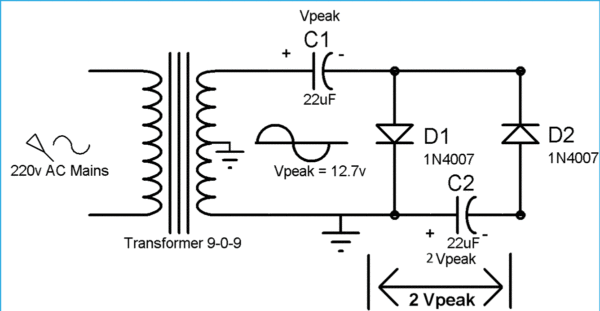# Fundamentals of Voltage Multiplier Notes for Electronics Engineering 1st Year

## What is Voltage Multiplier?

The Voltage Multiplier, however, is a special type of diode rectifier circuit which can potentially produce an output voltage many times greater than of the applied input voltage.

Voltage multipliers are similar in many ways to rectifiers in that they convert AC-to-DC voltages for use in many electrical and electronic circuit applications such as in microwave ovens, strong electric field coils for cathode-ray tubes, electrostatic and high voltage test equipment, etc, where it is necessary to have a very high DC voltage generated from a relatively low AC supply.

## Full Wave Voltage Multiplier:

The above circuit shows a basic symmetrical voltage multiplier circuit made up from two half-wave rectifier circuits. By adding a second diode and capacitor to the output of a standard half-wave rectifier, we can increase its output voltage by a set amount. This type of voltage multiplier configuration is known as a Full Wave Series Multiplier because one of the diodes is conducting in each half cycle, the same as for a full wave rectifier circuit.

## The Voltage Doubler:

A Voltage Doubler is a voltage multiplier circuit which has a voltage multiplication factor of two.

## Full wave Voltage Doubler:

As the name indicates, input voltage gets doubled through this circuit. The operation is Full wave voltage doubler is very simple: During the positive half cycle of

During the positive half cycle of Sinusoidal wave of AC, Diode D1 get forward biased and D2 get reversed biased, so capacitor C1 charges through the D1, to the peak value of sine wave (Vpeak). And during the negative half cycle of sine wave, D2 is forward biased and D1 revered biased, so capacitor C2 get charge through the D2, to Vpeak

## Half-Wave Voltage Doubler Circuit:During the first positive half cycle of Sinusoidal wave (AC), Diode D1 get forward biased and capacitor C1 get charged through the D1. Capacitor C1 get charged up to the peak voltage of AC i.e. Vpeak.

During the negative half cycle of the sine wave, Diode D2 conducts and D1 reverse biased. D1 blocks the discharging of capacitor C1. Now the capacitor C2 charge with the combined voltage of capacitor C1 (Vpeak) and the negative peak of the AC voltage that is also Vpeak. So the capacitor C2 charge up to 2Vpeak volt. Hence the voltage across capacitor C2 is two times the Vpeak of AC.

## Voltage Tripler Circuit:

A “voltage tripler circuit” consists of one and a half voltage doubler stages. This voltage multiplier circuit gives a DC output equal to three times the peak voltage value (3Vp) of the sinusoidal input signal.

As with the previous voltage doubler, the diodes within the voltage tripler circuit charge and block the discharge of the capacitors depending upon the direction of the input half-cycle. Then 1Vp is dropped across C3 and 2Vp across C2 and as the two capacitors are in series, this results in the load seeing a voltage equivalent to 3Vp.